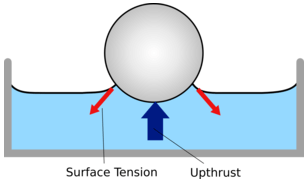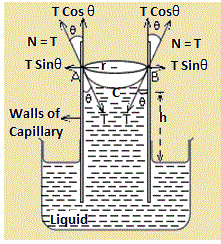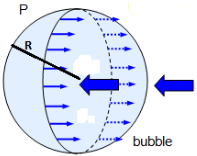NEET  >  Revision Notes: Liquids at Rest

# Revision Notes: Liquids at Rest Notes | Study NCERT Exemplar & Revision Notes for NEET - NEET

## Document Description: Revision Notes: Liquids at Rest for NEET 2022 is part of NCERT Exemplar & Revision Notes for NEET preparation. The notes and questions for Revision Notes: Liquids at Rest have been prepared according to the NEET exam syllabus. Information about Revision Notes: Liquids at Rest covers topics like and Revision Notes: Liquids at Rest Example, for NEET 2022 Exam. Find important definitions, questions, notes, meanings, examples, exercises and tests below for Revision Notes: Liquids at Rest.

Introduction of Revision Notes: Liquids at Rest in English is available as part of our NCERT Exemplar & Revision Notes for NEET for NEET & Revision Notes: Liquids at Rest in Hindi for NCERT Exemplar & Revision Notes for NEET course. Download more important topics related with notes, lectures and mock test series for NEET Exam by signing up for free. NEET: Revision Notes: Liquids at Rest Notes | Study NCERT Exemplar & Revision Notes for NEET - NEET
 1 Crore+ students have signed up on EduRev. Have you?

• Force of cohesion: It is force between two molecules of similar nature.
• Force of adhesion: It is the force between two molecules of different nature.
• Molecular range: The maximum distance between two molecules so that the force of attraction between them remains effective is called molecular range.
• Sphere of influence: Sphere of influence of any molecule is the sphere with molecule as its center and having a radius equal to molecular range (=10-7 cm).
• Surface film: Surface film of a liquid is defined as the portion of liquid lying on the surface and caught between two parallel planes situated molecular range apart.

Surface tension:Surface tension is the property of a liquid by virtue of which its free surface behaves like a stretched membrane and supports, comparatively heavier objects placed over it. It is measured in terms of force of surface tension.

Force of surface tension: It is defined as the amount of force acting per unit length on either side of an imaginary line drawn over the liquid surface.
(a) T = Force/length = F/l
(b) T = Surface energy/Surface area = W/A
Units: S.I – Nm-1
C.G.S- dyn cm-1

(a) For a cylindrical rod:- F = T×2πr  (Here r is the radius of cylindrical rod)
(b) For a rectangular block:- F = T×2(l+d) (Here l is the length and d is the thickness of the rectangular block)
(c) For a ring:- F = T×2×2πr  (Here r is the radius of cylindrical rod)

Surface energy: Potential energy per unit area of the surface is called surface energy.
(a) Expansion under isothermal condition: To do work against forces of surface tension:-
W= T × A    (Here A is the total increase in surface area)
To supply energy for maintaining the temperature of the film:-
E = T + H
(b) Expansion under adiabatic conditions:
E = T
Force of surface tension is numerically equal to the surface energy under adiabatic conditions.

DROPS AND BUBBLES
(a) Drop: Area of surface film of a spherical drop of radius R is given by, A = 4πR2
(b) Bubble: The surface area of the surface films of a bubble of radius R is, A = 2×4πR2

Combination of n drops into one big drop
(a) R = n1/3r
(b) E= n (4πr2T), Ef =4πR2T
(c) Ef/ Ei = n -1/3
(d) ΔE/E= [1-(1/n1/3)]
(e) ΔE = 4πR2T (n1/3-1) = 4πR3T (1/r – 1/R)

Angle of contact: Angle of contact,  for a pair of solid and liquid, is defined as the angle between tangent to the liquid surface drawn at the point of contact and the solid surface inside the liquid.
(a) When θ < 90º (acute):
Fa >Fc/√2
(i) Force of cohesion between two molecules of liquid is less than the force of adhesion between molecules of solid and liquid.
(ii) Liquid molecules will stick with the solid, thus making solid wet.
(iii) Such liquid is put in the solid tube; it will have meniscus concave upwards.
(b) When θ > 90º (obtuse):-Fa<Fc/√2
(i) Force of cohesion between two molecules of liquid is less than the force of adhesion between molecules of solid and liquid.
(ii) In this case, liquids do not wet the solids.
(iii) Such liquids when put in the solid tube will have a meniscus convex upwards.
(c) When θ  = 90º:-?
Fa=Fc/√2
The surface of liquid at the point of contact is plane. In this case force of cohesion and adhesion are comparable to each other.
(d) cosθc = Tsa – Tsl/Tla
Here, Tsa,Tsl and Tla represent solid-air, solid-liquid and liquid-air surface tension respectively). Here θc is acute if Tsl < Tsawhile θc is obtuse if Tsl >Tsa.

CapillarityCapillarity is the phenomenon, by virtue of which the level of liquid in a capillary tube is different from that outside it, is called capillarity.
Weight of liquid, W = Vρg = πr2[h+(r/3)]ρg    (Here r is the radius meniscus)
If weight of meniscus is taken into account, the force of surface tension will be,
T = [r(h+(r/3)) ρg]/2 cosθ
For fine capillary, force of surface tension, T = rhρg/2 cosθ
So height, h = 2T cosθ/ rρg
This signifies, height of liquid risen (or depressed) in a capillary tube varies inversely as the radius of tube. Smaller the diameter of capillary tube, greater is the rise of liquid in it.

Tube of insufficient length
Rh = 2T/ρg
As, T, ρ and g are all constant, Rh = Constant
Smaller the value of h, greater will be the value of R. But liquid will never flow.

Effect of temperature affecting surface tension of liquids
Surface tension of a liquid decreases with an increase in its temperature.
Tθ = K (θc-θ)
Here Tθ is the surface tension at a particular temperature θ while θc is the critical temperature of the liquid and K is constant.

Effect of density
Density of liquid also affects its surface tension. Surface tension of a liquid is given by,
T = A (ρ - ρ')n
Here, ρ is the density of liquid, ρ' is the density of saturated vapors of liquid and A is the constant depending on the nature of liquid.

Pressure difference across a liquid surface
(a) Plane surface: There is no difference of pressure on the two sides of the film.
(b) Convex surface: Pressure below the surface film must be greater than that just above it.
(c) Concave surface: Pressure on the upper side is greater than that just below it.

General formula for excess pressure
Pexcess =T[1/R1 + 1/R2]

• Excess pressure in liquid drop: Pexcess = 2T/R, Here R is the radius of liquid drop.
• Excess pressure for an air bubble in liquid drop: Pexcess = 2T/R• Excess pressure in soap bubble: Pexcess = 4T/R, Here R is the radius of soap bubble.
• Pressure inside an air bubble at a depth h in a liquid: Pin = Patm+ hdg + (2T/R)

Forces between two plates with thin water film separating them:
(a) ΔP = T (1/r – 1/R)
(b) F = ΔT (1/r – 1/R)
(c) If separation between plate is d, then  ΔP = 2T/d and  F = 2AT/d

Radius of curvature of common film: Rcomon = rR/R-r

Capillary depression, h = 2T cos (π-θ)/rdg

Shape of liquid surface
(a) Plane surface (as for water – silver) if Fadhesive > Fcohesive/√2
(b) Concave surface (as for water – glass) if Fadhesive > Fcohesive/√2
(c) Convex surface (as for mercury-glass) if Fadhesive < Fcohesive/√2

Increase in temperature: Δθ = 3T/ρs (1/r – 1/R) or Δθ = 3T/ρsJ (1/r – 1/R)

The document Revision Notes: Liquids at Rest Notes | Study NCERT Exemplar & Revision Notes for NEET - NEET is a part of the NEET Course NCERT Exemplar & Revision Notes for NEET.
All you need of NEET at this link: NEET

## NCERT Exemplar & Revision Notes for NEET

291 docs
 Use Code STAYHOME200 and get INR 200 additional OFF

## NCERT Exemplar & Revision Notes for NEET

291 docs

### How to Prepare for NEET

Read our guide to prepare for NEET which is created by Toppers & the best Teachers

Track your progress, build streaks, highlight & save important lessons and more!

,

,

,

,

,

,

,

,

,

,

,

,

,

,

,

,

,

,

,

,

,

;